# Test: Three Phase Induction Machine of Electrical Machines

## 10 Questions MCQ Test GATE Electrical Engineering (EE) 2023 Mock Test Series | Test: Three Phase Induction Machine of Electrical Machines

Description
Attempt Test: Three Phase Induction Machine of Electrical Machines | 10 questions in 30 minutes | Mock test for Electrical Engineering (EE) preparation | Free important questions MCQ to study GATE Electrical Engineering (EE) 2023 Mock Test Series for Electrical Engineering (EE) Exam | Download free PDF with solutions
QUESTION: 1

### A 3-phase, 50 Hz induction motor has a full load speed of 1440 rpm. The number of poles of the motor is

Solution: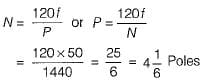Since the number of poles must be even and a whole number, therefore the number of poles must be 4.

QUESTION: 2

### A properly shunted centre-zero galvanometer is connected in the rotor circuit of a 6-pole, 50 Hz would-rotor induction motor. If the galvanometer makes 90 complete oscillations in one minute, the speed of rotor would be

Solution:

Rotor frequency,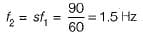∴ Slip,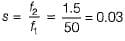So, rotor speed,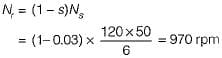QUESTION: 3

### A 10-pole, 3-phase, 50 Hz, delta connected induction motor takes 120 kW during blocked-rotor test at rated voltage and frequency. If the stator ohmic loss is equal to rotor ohmic loss during blocked rotor test, then the starting torque if DOL starting is used for this motor will be

Solution:

During blocked rotor test, power input to a 3-phase induction motor appears almost entirely as I2R loss in both stator and rotor windings. Since, stator ohmic loss = rotor ohmic loss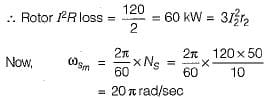So, starting torque,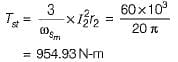QUESTION: 4

A 3-phase squirrel cage induction motor has maximum torque equal to twice the full-load torque. The per phase rotor resistance and per phase standstill reactance referred to stator are 0.2 Ω and 2 Ω respectively (Stator impedance is neglected). What is the ratio of motor starting toque to its full-load torque, if the motor is started by direct-on-line starter?

Solution: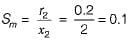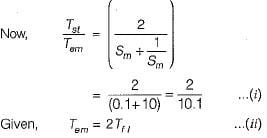From equations (i) and (ii), we have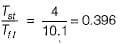QUESTION: 5

A 10 kW, 400 V, 3-phase induction motor draw a current of 5 times its full-load current at rated voltage and at standstill developing a starting toque of 1.5 times its full-load torque. An autotransformer is installed to reduce the starting current and to give full-load torque at starting. Neglecting exciting current of auto-transformer, the voltage applied to the motor terminals at the time of starting with auto transformer is

Solution: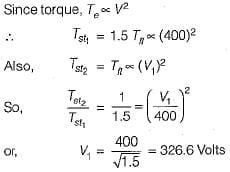QUESTION: 6

A 3-phase, 20 kW, 400 V, 1470 rpm, 50 Hz squirrel cage induction motor develops a torque of 100 N-m at a speed of 1400 rpm. if the motor is connected to a 30 Hz supply; for keeping the same air-gap flux, the supply voltage and the speed for the same load torque would respectively be

Solution:

For same air-gap flux,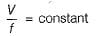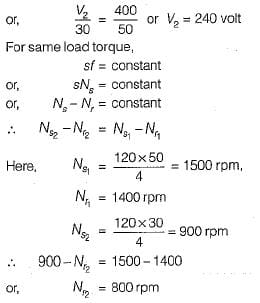QUESTION: 7

In a 3-phase squirrel cage induction motor, applied voltage is reduced to x times its rated value. Then, its starting line current from mains and starting torque by auto-transformer starting would reduce respectively from their corresponding direct switching values by a factor of

Solution:
QUESTION: 8

A 3-phase IM with stator in delta draws a line current of 10 A. When connected in star, it would draw a line current of

Solution: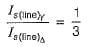or,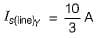QUESTION: 9

The power factor of a delta-connected 3-phase, 50 kW, IM is 0.4 when delivering 35% of its rated load. If the stator is reconnected in star, then its

Solution:

We know that,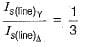∴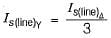Thus, stator current will decrease.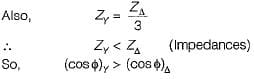QUESTION: 10

A 3-phase, star-connected SRIM is fed from 400 V, 50 Hz source. Stator to rotor effective turns ratio is 2. At a rotor speed of 1440 rpm, the rotor induced emf per phase would be

Solution: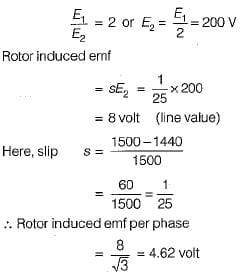Use Code STAYHOME200 and get INR 200 additional OFF Use Coupon Code